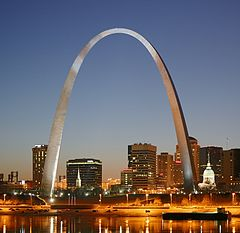Mahesh Godavarti
2I will talk about quadratic functions y = ax^2 + bx + c. If you graph this the shape you would get is a parabola. A quadratic equation is just a special case of a quadratic function where y = 0 . A famous example of application of quadratic functions is the Gateway Arch in St. Louis. For it to be structurally sound, it has to be shaped like a parabola - a quadratic function! Image source - Wikipedia